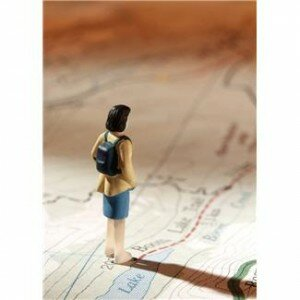# Drawing with Pythagoras“Pythagorean Theorem?!!”…I know what you are thinking…..(You can say to your class)…..”OMG  Teacher…..What could we possibly ever use this for, in the future of our lives?“……Well, in this article, we talk about real life examples of using the Pythagorean Theorem.  First let’s learn a basic calculation, corresponding to the diagram here to the <<left, followed by a bit of Pythagorean history.  The basis of the theorem is that the Area of the 2 Squares of the 2 Lines that form the Right Angle of a Right Triangle are the equivalent ( = ) Area of the Square that opposes them in the Triangle. If you look at the diagram here to the << left, the Areas of “a” added to “b” = the Area of “c” (the Square of the opposite line (or “leg”). That opposite line (or  “c” “leg”) is called the hypotenuse. Also, if one of the lines “a” of the right triangle is 4 inches and the other line “b”  is 6 inches, we can calculate how long the hypotenuse is, or the “third leg”. Letting a = 4, b=6, and c= the length of the hypotenuse.  (4)^2 + (6)^2 = c^2. Accordingly, 4 x 4 =16, and 6 x 6 = 36. Thus, 16 + 36 = 52. The square root of 52 is approximately 7.21 , hence the length of the hypotenuse or “third leg“ of the right triangle, is 7.21 inches.

The Pythagorean Theorem is named after the Greek mathematician Pythagoras. Many believe the first discovery and proving of this ancient math theorem came before Pythagoras, but since no tangible account of this has yet been documented, it is named as such. If that was true, however, we wonder what another name of the theorem would have been, and from what country and nation? (a fun question to ponder).  The Pythagorean Theorem can be used with any shape and for any formula that squares a number. And, in fact, the area of any shape can be computed from any line segment squared.  http://en.wikipedia.org/wiki/Pythagorean_theorem  (reference also for Diagram Above).   Apparently, even the teenage Brainiac Lisa Simpson from The Simpsons television series knows all about Pythagoras.  On this site, there are some cool diagrams showing the differences of Lines (Segments / Legs), Radius, and Area: http://betterexplained.com/articles/surprising-uses-of-the-pythagorean-theorem/.  And, to assist in figuring out these math scenarios, this site offers tabs for entering in numbers to calculate the square root of the numbers in question: http://www.math.com/students/calculators/source/square-root.html.Finally, here are a few Real Life Examples:

Meet Me at The Corner:
Let’s say Stephanie and Maria are meeting at the Hiking Trail Entrance on the corner of Saanich Rd. and Cedar Rd. One phones the other on her mobile phone and asks, “How long will it be before you arrive at the entrance?”… “Well, let’s estimate by first finding out how far away we are from each other.” In present time, Stephanie is on Saanich Rd. to and is 10 miles away. Meanwhile, Maria is on Cedar Rd. and is 4 miles away.  How far away from one another are Stephanie and Maria?  The distance between them = a^2 + b^2 = c^2 or, respectively: 10^2 (10 squared) + 4^2 (4 squared); or respectively, 100 +16 = 116 miles. The square root of 116 is 10.77. Thus, Stephanie and Maria are 10.77, almost 11 miles away from one another. Hence, they figure they will be another hour on their bicycles to meet one another to go hiking.

Firefighters Needing to Know Height of a Building:
3 Firefighters receive a call to help Ann rescue her cat Tia from the Oak Tree outside her window. The tree is about 3 stories tall, and the Tia, after chasing a squirrel, is stuck on a branch at about the height of 2 stories of her house. The height to the branch may be 20 feet, and the firefighters have to put the ladder about 10 feet away from the Oak Tree in order to go around Ann‘s shed. How long of a ladder do the firefighters need in order to rescue Ann‘s cat? a^2 + b^2 = c^2 or, respectively: (20)^2 + (10)^2 = 2^2, the length of ladder required. 400 + 100 = 500. The square root of 500 is approximately 22.37. The firefighters extend their expandable ladder to be approximately 23 feet, whereas they need at least 22.37 feet to safely reach the Oak branch. Ann’s cat Tia is rescued and All are Happy!

For more fun and interesting Learning Math Games, you can visit us here:
http://www.math-lessons.ca/activities/FractionsBoard5.html
http://www.math-lessons.ca/timestables/times-tables.html
http://www.math-lessons.ca/activities/FractionsBoard4.htmlhttp://www.math-lessons.ca/index.html